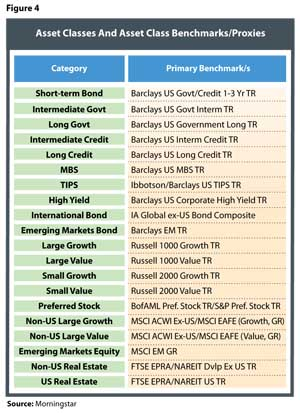# Efficient Income Investing

December 21, 2012

Before continuing, it is important to note that the relative components for an efficient total return portfolio can be decomposed into an income return and a price return. Given the relative risk and return of attributes typically included in a portfolio, as one moves “up” the efficient frontier the portfolio tends to focus more heavily on price return than income return, which dominates the more conservative portfolios. The concept is illustrated in Figure 2.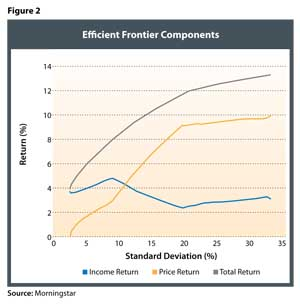The frontier in Figure 2 (the green line) is the traditional mean-variance efficient frontier that is created by maximizing total return and minimizing variance. The proportion of return generated by income is not specified. The income return (blue line) and price return (red line) are simply the component parts of the portfolio’s total return. If we change the definition of “optimal” to focus on income return1 only, we get a different efficient frontier, which lies below the total return efficient frontier, as depicted in Figure 3.Note that the income return frontier in figure 3 is truncated. It only extends as far as the highest yielding investment which, in this case, is US preferred stock. An investor seeking income greater than this amount (7.5 percent) would need to liquidate some of the portfolio (i.e., dip into the Price Return) to achieve a given consumption objective. However, we will focus on the differences in the portfolio allocations that fall within the same total return range and demonstrate the considerable differences that result from the different allocation methodologies.

The Income Return Utility Function

The traditional mean-variance utility function, as noted in equation one (below), is focused on maximising the total return for a given unit of risk: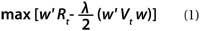Where:

• w = an N vector of asset class weights to be solved for
Rt = an N vector of total returns
λ = a aversion coefficient
Vt = an N by N total return covariance matrix

In order to determine the optimal income return portfolio we rewrite (1) to separate income from total return. We could, of course, separate income return from price return, but for an income-oriented investor, the total return should represent the “minimum-income frontier.” In other words, an income investor would never want less income than that generated by the total return frontier.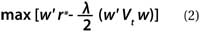For r*: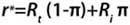Where:

• w = an N vector of asset class weights to be solved for
λ = a risk aversion coefficient
Vt = an N by N total return covariance matrix
Rt = an N vector of total returns
Ri = an N vector of income returns (yields)
π = preference for income return (yield) defined on the [0,1] interval

Critically, the risk component in the income return function (equation 2) is not based solely on income risk (i.e., the income return covariance matrix). Income investors without a fixed time horizon are very sensitive to total return risk which can be substantial. For example, while the standard deviation of the income return from small value has historically been relatively low, there is a significant amount of potential risk associated with the price return. The approach introduced in this paper, therefore, uses total return risk as the relevant risk measure. We also assume an income preference of 1 across the efficient frontier.

Estimating Total Return

For our analysis, we include the asset classes listed in Figure 4. Significantly, we don’t limit the opportunity set to higher-yielding asset classes since our goal is to strike a balance between total return, total risk, and income. The only arguably non-core asset class we include is preferred stock which, given the yield focus, cannot be ignored.